# Estimating Simple Models from Real Laboratory Process Data

This example shows how to develop and analyze simple models from a real laboratory process data. We start with a small description of the process, learn how to import the data to the toolbox and preprocess/condition it and then proceed systematically to estimate parametric and nonparametric models. Once the models have been identified we compare the estimated models and also validate the model to the actual output data from the experiment.

### System Description

This case study concerns data collected from a laboratory scale "hairdryer". (Feedback's Process Trainer PT326; See also page 525 in Ljung, 1999). The process works as follows: Air is fanned through a tube and heated at the inlet. The air temperature is measured by a thermocouple at the outlet. The input is the voltage over the heating device, which is just a mesh of resistor wires. The output is the outlet air temperature represented by the measured thermocouple voltage.

### Setting up Data for Analysis

First we load the input-output data to the MATLAB® Workspace.

```load dryer2; ```

Vector `y2`, the output, contains 1000 measurements of the thermocouple voltage which is proportional to the temperature in the outlet airstream. Vector `u2` contains 1000 input data points consisting of the voltage applied to the heater. The input was generated as a binary random sequence that switches from one level to the other with probability 0.2. The sample time is 0.08 seconds.

The next step is to set up the data as an iddata object

```dry = iddata(y2,u2,0.08); ```

To get information about the data, just type the name of the `iddata` object at the MATLAB command window:

```dry ```
```dry = Time domain data set with 1000 samples. Sample time: 0.08 seconds Outputs Unit (if specified) y1 Inputs Unit (if specified) u1 ```

To inspect the properties of the above iddata object, use the `get` command:

```get(dry) ```
```ans = struct with fields: Domain: 'Time' Name: '' OutputData: [1000x1 double] y: 'Same as OutputData' OutputName: {'y1'} OutputUnit: {''} InputData: [1000x1 double] u: 'Same as InputData' InputName: {'u1'} InputUnit: {''} Period: Inf InterSample: 'zoh' Ts: 0.0800 Tstart: [] SamplingInstants: [1000x0 double] TimeUnit: 'seconds' ExperimentName: 'Exp1' Notes: {} UserData: [] ```

For better book-keeping, it is good practice to give names to the input and output channels and Time units. These names would be propagated throughout the analysis of this iddata object:

```dry.InputName = 'Heater Voltage'; dry.OutputName = 'Thermocouple Voltage'; dry.TimeUnit = 'seconds'; dry.InputUnit = 'V'; dry.OutputUnit = 'V'; ```

Now that we have the data set ready, we choose the first 300 data points for model estimation.

```ze = dry(1:300) ```
```ze = Time domain data set with 300 samples. Sample time: 0.08 seconds Outputs Unit (if specified) Thermocouple Voltage V Inputs Unit (if specified) Heater Voltage V ```

### Preprocessing the Data

Plot the interval from sample 200 to 300:

```plot(ze(200:300)); ```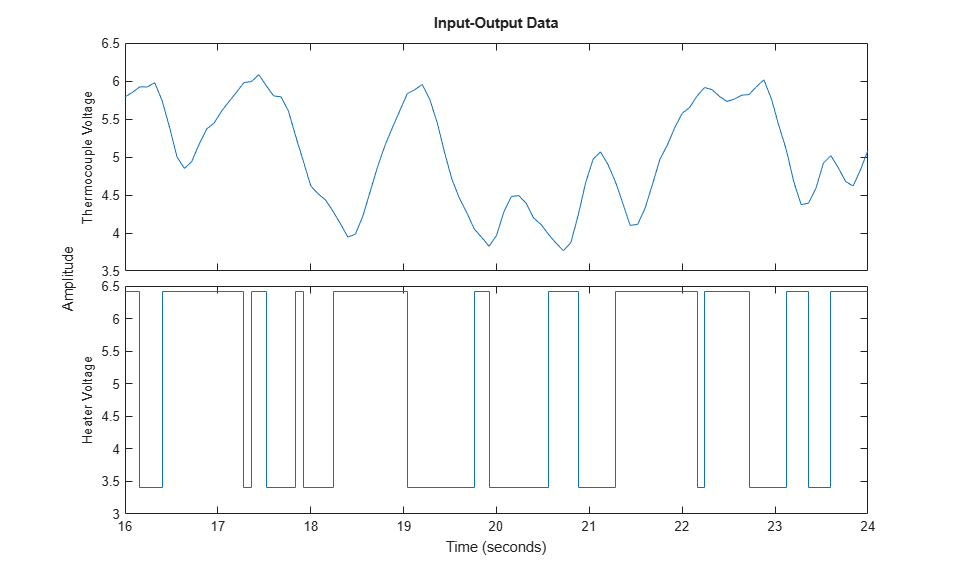Figure 1: A snapshot of the measured hair-dryer data.

From the above plot, it can be observed that the data is not zero mean. So let us remove the constant levels and make the data zero mean.

```ze = detrend(ze); ```

The same data set after it has been detrended:

```plot(ze(200:300)) %show samples from 200 to 300 of detrended data ```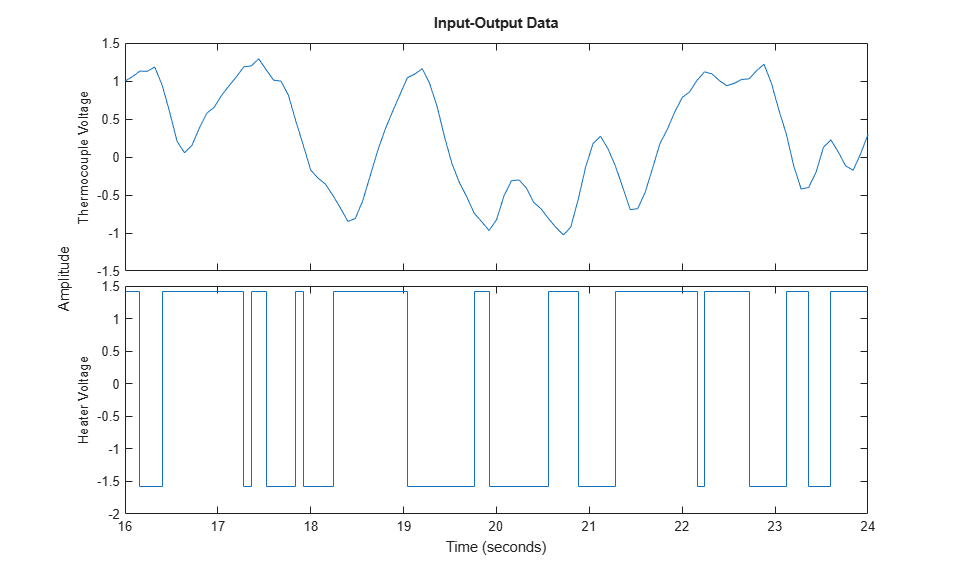Figure 2: Detrended estimation data.

### Estimating Nonparametric and Parametric Models

Now that the dataset has been detrended and there are no obvious outliers, let us first estimate the impulse response of the system by correlation analysis to get some idea of time constants and the like:

```clf mi = impulseest(ze); % non-parametric (FIR) model showConfidence(impulseplot(mi),3); %impulse response with 3 standard %deviations confidence region ```Figure 3: Impulse response of the FIR model estimated using `ze`.

The shaded region marks a 99.7% confidence interval. There is a time delay (dead-time) of 3 samples before the output responds to the input (significant output outside the confidence interval).

The simplest way to get started on a parametric estimation routine is to build a state-space model where the model-order is automatically determined, using a prediction error method. Let us estimate a model using the `ssest` estimation technique:

```m1 = ssest(ze); ```

`m1` is a continuous-time identified state-space model, represented by an `idss` object. The estimation algorithm chooses 3 as the optimal order of the model. To inspect the properties of the estimated model, just enter the model name at the command window:

```m1 ```
```m1 = Continuous-time identified state-space model: dx/dt = A x(t) + B u(t) + K e(t) y(t) = C x(t) + D u(t) + e(t) A = x1 x2 x3 x1 -0.4839 -2.011 2.092 x2 3.321 -1.913 5.998 x3 1.623 -17.01 -15.61 B = Heater Volta x1 -0.05753 x2 0.02004 x3 1.377 C = x1 x2 x3 Thermocouple -14.07 0.07729 0.04252 D = Heater Volta Thermocouple 0 K = Thermocouple x1 -0.9457 x2 -0.02097 x3 2.102 Parameterization: FREE form (all coefficients in A, B, C free). Feedthrough: none Disturbance component: estimate Number of free coefficients: 18 Use "idssdata", "getpvec", "getcov" for parameters and their uncertainties. Status: Estimated using SSEST on time domain data "ze". Fit to estimation data: 95.32% (prediction focus) FPE: 0.001621, MSE: 0.001526 ```

The display suggests that the model is free-form (all entries of A, B and C matrices were treated as free parameters) and that the estimated model fits the data pretty well (over 90% fit). To retrieve the properties of this model, for example to obtain the `A` matrix of the discrete state-space object generated above, we can use the dot operator:

` A = m1.a;`

See the "Data and Model Objects in System Identification Toolbox" example for more information regarding model objects. To find out which properties of the model object can be retrieved, use `get` command:

```get(m1) ```
``` A: [3x3 double] B: [3x1 double] C: [-14.0706 0.0773 0.0425] D: 0 K: [3x1 double] StateName: {3x1 cell} StateUnit: {3x1 cell} Structure: [1x1 pmodel.ss] NoiseVariance: 1.2587e-04 Report: [1x1 idresults.ssest] InputDelay: 0 OutputDelay: 0 Ts: 0 TimeUnit: 'seconds' InputName: {'Heater Voltage'} InputUnit: {'V'} InputGroup: [1x1 struct] OutputName: {'Thermocouple Voltage'} OutputUnit: {'V'} OutputGroup: [1x1 struct] Notes: [0x1 string] UserData: [] Name: '' SamplingGrid: [1x1 struct] ```

To fetch the values of the state-space matrices and their 1 standard deviation uncertainties, use the `idssdata` command:

```[A,B,C,D,K,~,dA,dB,dC,dD,dK] = idssdata(m1) ```
```A = -0.4839 -2.0112 2.0917 3.3205 -1.9135 5.9981 1.6235 -17.0096 -15.6070 B = -0.0575 0.0200 1.3770 C = -14.0706 0.0773 0.0425 D = 0 K = -0.9457 -0.0210 2.1019 dA = 1.0e+15 * 0.1587 0.0982 0.1185 0.3280 0.2625 0.2261 1.2869 0.8385 0.2834 dB = 1.0e+13 * 0.6091 1.7606 6.0663 dC = 1.0e+14 * 2.9843 1.9520 0.6506 dD = 0 dK = 1.0e+14 * 0.1911 0.5194 1.0667 ```

The uncertainties are quite large even though the model fit the estimation data well. This is because the model is over-parameterized, that is, it has more free parameters than what could be uniquely identified. The variance of parameters in such cases is not well defined. However this does not imply that the model is unreliable. We can plot the time- and frequency-response of this plot and view the variance as confidence regions as discussed next.

### Analyzing the Estimated Model

The Bode plot of the generated model can be obtained using the `bode` function as shown below:

```h = bodeplot(m1); ```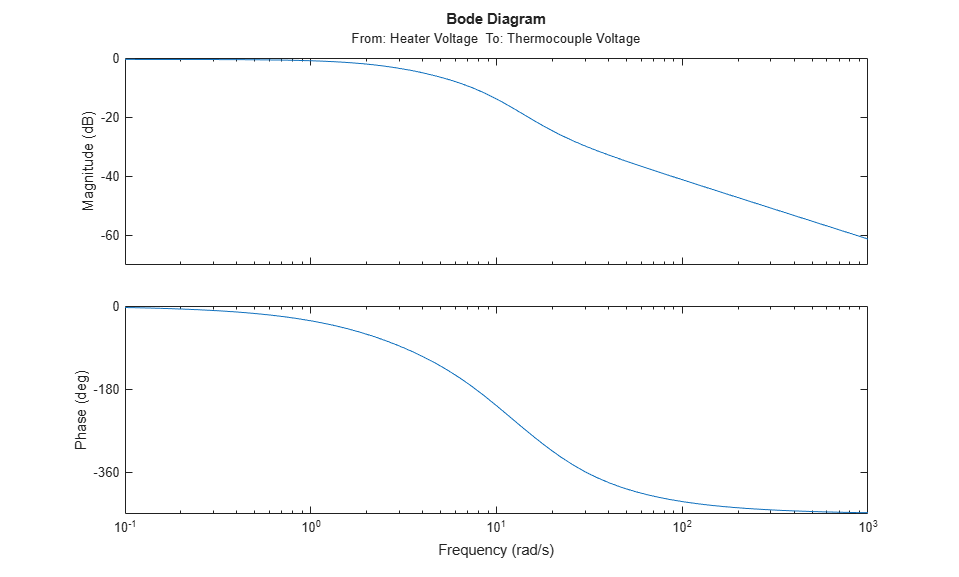Figure 4: Bode plot of estimated model.

Right-click on the plot and pick Characteristics->Confidence Region. Or, use the `showConfidence` command to view the variance of the response.

```showConfidence(h,3) % 3 standard deviation (99.7%) confidence region ```Figure 5: Bode plot with 3 standard deviation confidence region.

Similarly, we can generate the step plot and its associated 3 standard deviation confidence region. We can compare the responses and associated variances of the parametric model `m1` with that of the nonparametric model `mi`:

```showConfidence(stepplot(m1,'b',mi,'r',3),3) ```Figure 6: Step plot of models `m1` and `mi` with confidence regions.

We can also consider the Nyquist plot, and mark uncertainty regions at certain frequencies with ellipses, corresponding to 3 standard deviations:

```Opt = nyquistoptions; Opt.ShowFullContour = 'off'; showConfidence(nyquistplot(m1,Opt),3) ```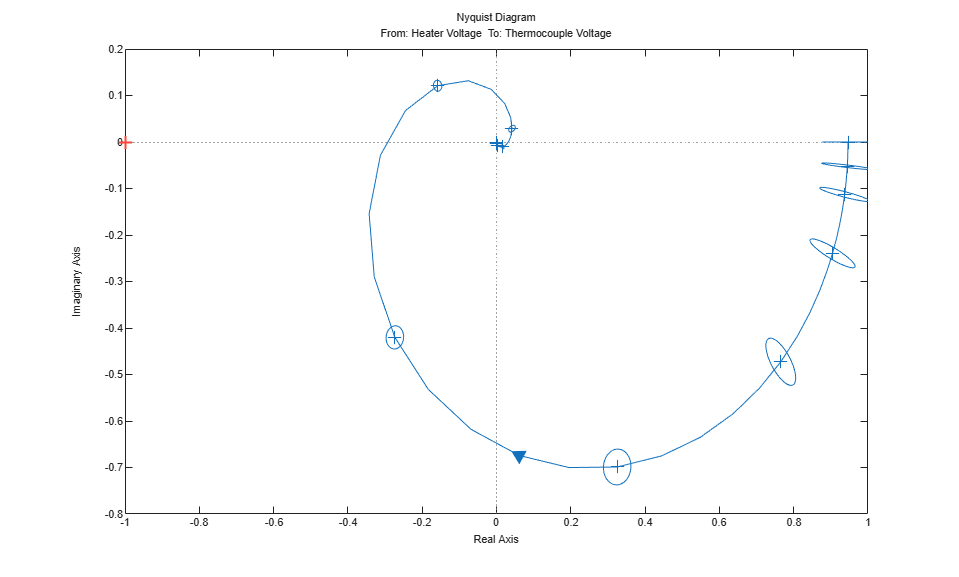Figure 7: Nyquist plot of estimated model showing the uncertainty regions at certain frequencies.

The response plots show that the estimated model `m1` is quite reliable.

### Estimating Models with a Prescribed Structure

System Identification Toolbox can also be used to obtain a model with a prescribed structure. For example, a difference equation model with 2 poles, 1 zero and 3 sample delays can be obtained using the `arx` function as shown below:

```m2 = arx(ze,[2 2 3]); ```

To look at the model, enter the model name at the command window.

```m2 ```
```m2 = Discrete-time ARX model: A(z)y(t) = B(z)u(t) + e(t) A(z) = 1 - 1.274 z^-1 + 0.3942 z^-2 B(z) = 0.06679 z^-3 + 0.04429 z^-4 Sample time: 0.08 seconds Parameterization: Polynomial orders: na=2 nb=2 nk=3 Number of free coefficients: 4 Use "polydata", "getpvec", "getcov" for parameters and their uncertainties. Status: Estimated using ARX on time domain data "ze". Fit to estimation data: 95.08% (prediction focus) FPE: 0.001756, MSE: 0.001687 ```

A continuous time transfer function with 2 poles, one zero and 0.2 second transport delay can be estimated using the `tfest` command:

```m3 = tfest(ze, 2, 1, 0.2) ```
```m3 = From input "Heater Voltage" to output "Thermocouple Voltage": 1.183 s + 26.55 exp(-0.2*s) * --------------------- s^2 + 11.61 s + 28.63 Continuous-time identified transfer function. Parameterization: Number of poles: 2 Number of zeros: 1 Number of free coefficients: 4 Use "tfdata", "getpvec", "getcov" for parameters and their uncertainties. Status: Estimated using TFEST on time domain data "ze". Fit to estimation data: 88.79% FPE: 0.009126, MSE: 0.008768 ```

### Validating the Estimated Model to Experimental Output

How good is an estimated model? One way to find out is to simulate it and compare the model output with measured output. Select a portion of the original data that was not used in building the model, say from samples 800 to 900. Once the validation data has been preprocessed, we use the `compare` function as shown below to view the quality of prediction:

```zv = dry(800:900); % select an independent data set for validation zv = detrend(zv); % preprocess the validation data set(gcf,'DefaultLegendLocation','best') compare(zv,m1); % perform comparison of simulated output ```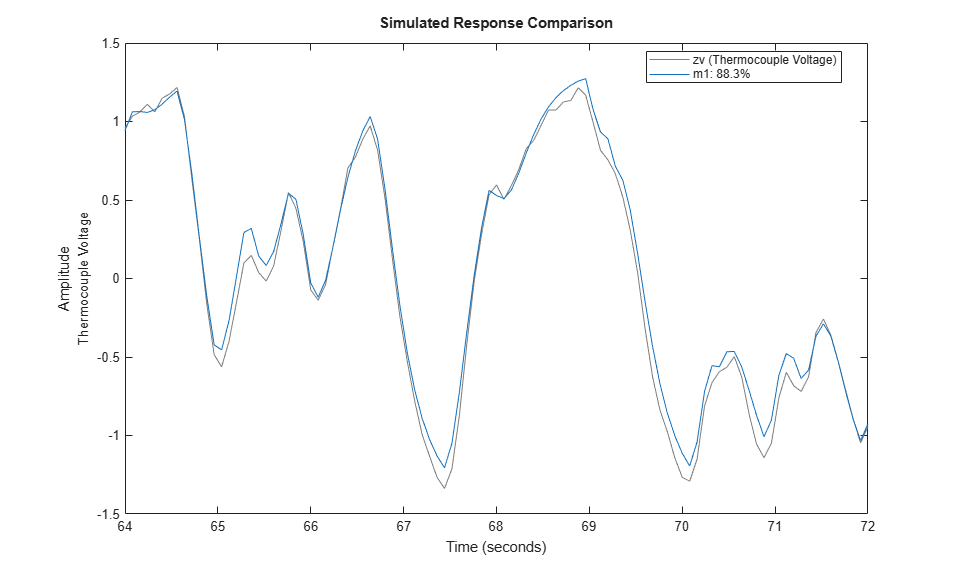Figure 8: Model's simulated response vs. validation data output.

It can be observed here that the agreement is very good. The "Fit" value shown is calculated as:

`Fit = 100*(1 - norm(yh - y)/norm(y-mean(y)))`

where `y` is the measured output (=|zv.y|), and `yh` is the output of the model `m1`.

### Comparing Estimated Models

To compare the performance of the models that we have estimated, for example `m1`, `m2` and `m3` with the validation data `zv`, we can again use the `compare` command:

```compare(zv,m1,'b',m2,'r',m3,'c'); ```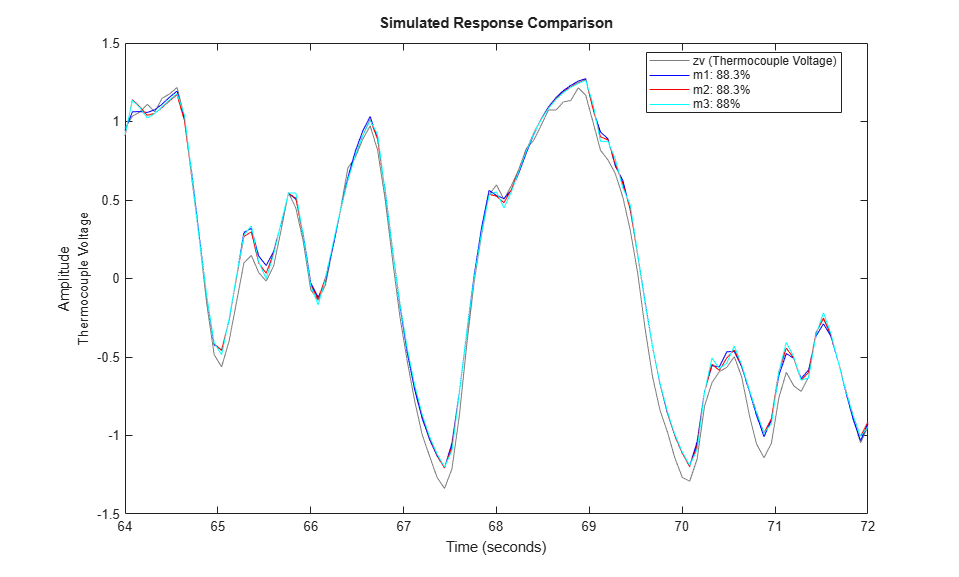Figure 9: Comparing the responses of models `m1`, `m2`, `m3` on validation data set `ze`.

The pole-zero plots for the models can be obtained using `iopzplot`:

```h = iopzplot(m1,'b',m2,'r',m3,'c'); ```Figure 10: Poles and zeros of the models `m1`, `m2` and `m3`.

The uncertainties in the poles and zeroes can also be obtained. In the following statement, '3' refers to the number of standard deviations.

```showConfidence(h,3); ```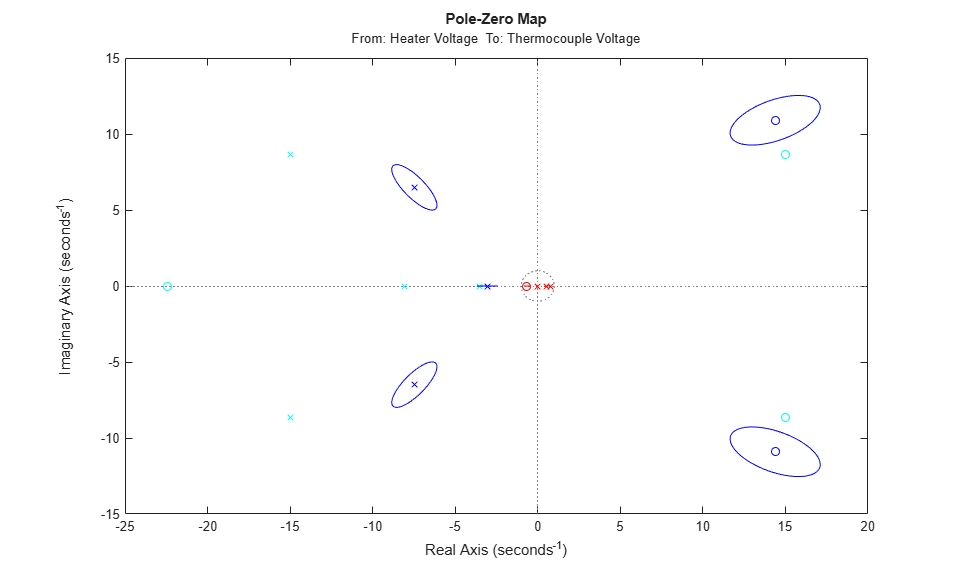Figure 11: Pole-zero map with uncertainty regions.

The frequency functions above that are obtained from the models can be compared with one that is obtained using a non-parametric spectral analysis method (`spa`):

```gs = spa(ze); ```

The `spa` command produces an IDFRD model. The `bode` function can again be used for a comparison with the transfer functions of the models obtained.

```w = linspace(0.4,pi/m2.Ts,200); opt = bodeoptions; opt.PhaseMatching = 'on'; bodeplot(m1,'b',m2,'r',m3,'c',gs,'g',w,opt); legend('m1','m2','m3','gs') ```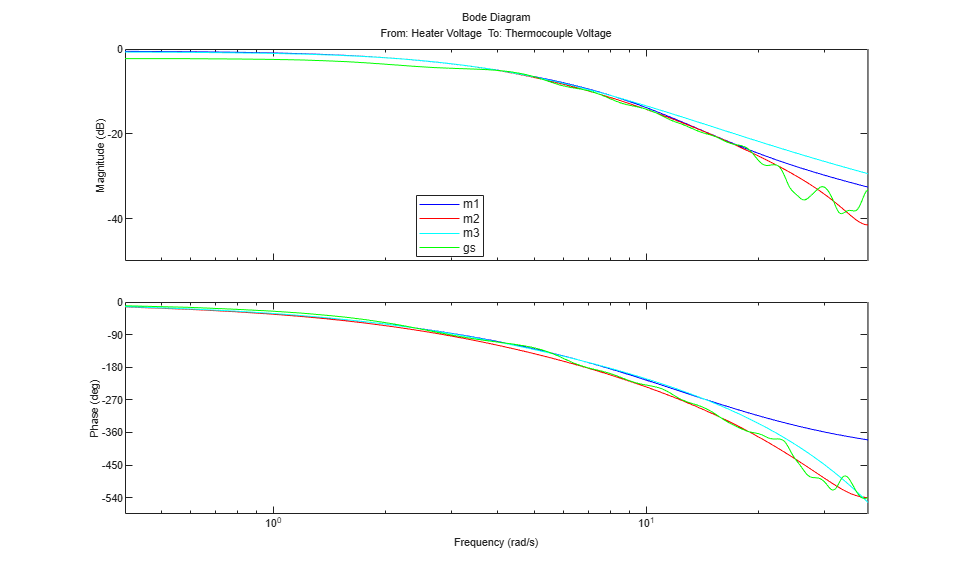Figure 12: Bode responses of `m1`, `m2` and `m3` compared against the non-parametric spectral estimation model `gs`.

The frequency responses from the three models/methods are very close. This indicates that this response is reliable.

Also, a Nyquist plot can be analyzed with the uncertainty regions marked at certain frequencies:

```showConfidence(nyquistplot(m1,'b',m2,'r',m3,'c',gs,'g'),3) ```Figure 13: Nyquist plots of models `m1`, `m2`, `m3` and `gs`.

The non-parametric model `gs` exhibits the most uncertainty in response.